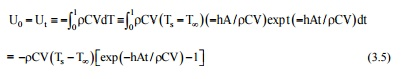Home | | Heat and Mass Transfer | Solved Problems - Heat and Mass Transfer - Conduction

# Solved Problems - Heat and Mass Transfer - Conduction

Mechanical - Heat and Mass Transfer - Conduction

1. A composite wall consists of three layers of thicknesses 300 mm, 200mm and100mm with thermal conductivities 1.5, 3.5 and is W/m K respectively. The inside surface is exposed to gases at 1200°C with convection heat transfer coefficient as 30W/m2K. The temperature of air on the other side of the wall is 30°C with convective heat transfer coefficient 10 Wm2K. If the temperature at the outside surface of the wall is 180°C, calculate the temperature at other surface of the wall, the rate of heat transfer and the overall heat transfer coefficient.

Solution: The composite wall and its equivalent thermal circuits is shown in the figure.2.Derivethe General Heat Conduction Equation for an Isotropic Solid with   Constant

Thermal Conductivity in Cartesian coordinates.

Any physical phenomenon is generally accompanied by a change in space and time of its physical properties. The heat transfer by conduction in solids can only take place when there is a variation of temperature, in both space and time. Let us consider a small volume of a solidIt should be noted that Fourier law can always be used to compute the rate of heat transfer by conduction from the knowledge of temperature distribution even for unsteady condition and with internal heat generation.

A 20 cm thick slab of aluminums (k = 230 W/mK) is placed in contact with a 15 cm thick stainless steel plate (k = 15 W/mK). Due to roughness, 40 percent of the area is in direct contact and the gap (0.0002 m) is filled with air (k = 0.032 W/mK). The difference in temperature between the two outside surfaces of the plate is 200°C Estimate (i) the heat flow rate, (ii) the contact resistance, and (iii) the drop in temperature at the interface.

Solution: Let us assume that out of 40% area m direct contact, half the surface area is occupied by steel and half is occupied by aluminums.

The physical system and its analogous electric circuits is shown in Fig. 1.3.4. A steel pipe. Inside diameter 100 mm, outside diameter 120 mm (k 50 W/m K) IS Insulated with a40mm thick hightemperature Insulation(k = 0.09 W/m K) and another Insulation 60 mm thick (k = 0.07 W/m K). The ambient temperature IS 25°C. The heattransfer coefficient for the inside and outside surfaces are 550 and 15 W/m2K respectively. The pipe carries steam at 300oC. Calculate (1) the rate of heat loss by steam per unit length of the pipe (11) the temperature of the outside surface .

Solution: A cross-section of the pipe with two layers of insulation is shown Fig. 1.4with its analogous electrical circuit.Fig1.4A crosssection through an insulated cylinder, thermal resistances in series.

For L = 1.0 m.5. Steel balls 10 mm in diameter (k = 48 W/mK), (C = 600 J/kgK) are cooled in air at temperature 35°C from an initial temperature of 750°C. Calculate the time required for the temperature to drop to 150°C when h = 25 W/m2K and density p = 7800 kg/m3. (AU 2012).

Solution: Characteristic length, L = VIA = 4/3 pr3/4 pr2 = r/3 = 5 × 10-3/3m Bi = hL/k = 25 × 5 × 10-3/ (3 × 48) = 8.68 × 10-4<< 0.1,

Since the internal resistance is negligible, we make use of lumped capacity analysis: Eq. (3.4),

( T -T¥) / ( Ts -T¥)=exp(-Bi Fo) ; (150 35) / (750 35) = 0.16084

\Bi × Fo = 1827; Fo = 1.827/ (8. 68 × 10-4) 2.1× 103

Or, at/ L2 = k/ ( rCL2)t = 2100 and t = 568 = 0.158 hour

We can also compute the change in the internal energy of the object as:= -7800 × 600 × (4/3) p(5 × 10-3)3 (750-35) (0.16084 - 1)

= 1.47 × 103 J = 1.47 kJ.

If we allow the time't' to go to infinity, we would have a situation that corresponds to steady state in the new environment. The change in internal energy will be U0 - U¥ = [ rCV( Ts -T¥) exp(-

¥)- 1] = [ rCV( Ts -T¥].

We can also compute the instantaneous heal transfer rate at any time.

Or. Q = - rVCdT/dt = - rVCd/dt[ T¥+ ( Ts -T¥ )exp(-hAt/ rCV) ]

= hA( Ts -T¥)[exp(-hAt/ rCV)) and for t = 60s,

Q = 25 × 4 × 3.142 (5 × 10-3)2(750 35) [exp( -25 × 3 × 60/5 × 10-3 × 7800 × 600)] = 4.63 W.

6.Aluminums fins 1.5 cm wide and 10 mm thick are placed on a 2.5 cm diameter tube to dissipate the heat. The tube surface temperature is 170°C ambient temperatures is 20°C. calculate the heat loss per fin. Take h = 130 W/m2 C and K = 200 W/m2 C for aluminums.

Given

Wide of the fin b = 1.5 cm = 1.5 ´10-2 m

Thickness t = 10 mm = 10 ´10-3 m

Diameter of the tube d = 2.5 cm = 2.5 ´10-2 m

Surface temperature Tb = 170°C + 273 = 443 K

Ambient temperature T¥ = 20°C + 273 = 293 K

Heat transfer co-efficient h = 130 W/m2°C

Thermal conductivity K = 200 W/m°C

Solution

Assume fin end is insulated, so this is short fin end insulated type problem.

Heat transfer [short fin, end insulated]

Q = (hPKA)1/2 (Tb - T¥) tan h(mL)   ……..(1)   [FromNoHMT.41]   data   book

Where